# How to calculate differences between dates in Google SheetsFrom basic functions to the most advanced formulas, Google Sheets is a staple for many users managing data. This includes calculating the difference between dates, too. There are a variety of situations where calculating the difference between dates is an essential task for business operations. An example of this is in project management. You may need to calculate the duration of entire projects in order to delegate these to your team members. In this article, we will show you how to calculate the difference between dates in Google Sheets. This will help to streamline your process and maximize your overall efficiency.

### Calculating the differences between two dates in Google Sheets

Google Sheets offers several options in which you can calculate your dates. Each varies in the level of complexity to suit your spreadsheet experience or objectives. Let’s first look at the simpler ways you can calculate the difference between dates in Google Sheets.

### How to subtract dates in Google Sheets easily

#### Option 1: Simply subtract

Firstly, it’s important to note that Google Sheets will process dates exactly as any other numerical value. In other words, you can use simple calculations like add and subtract to calculate the difference between your two dates. So, the simplest way is to type a simple minus (-) between your dates.

Simply input: =[end_date]-[start_date]

=[end_date]-[start_date]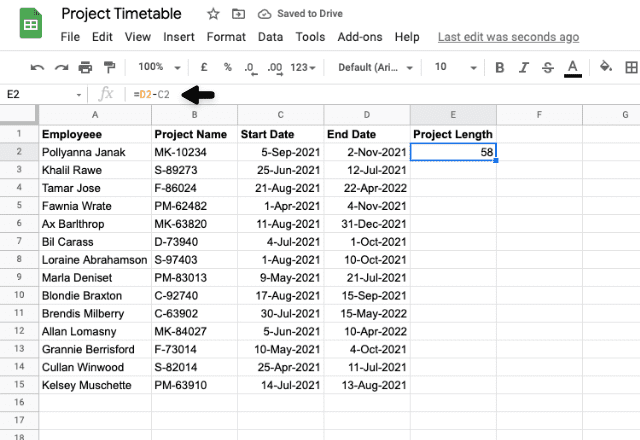Please note that when you subtract dates, Google Sheets does not include the start date in the total. For example, let’s say your project starts on 5 May and ends on 12 May. Using a subtract calculation, the result is 7. It counts 7 days: 6th, 7th, 8th, 9th, 10th, 11th, and 12th May.

So, if you want to include the start date, you must add 1 to the end of your formula:

=[end_date]-[start_date]+1

=[end_date]-[start_date]+1

You can also use the MINUS function in the same way. Simply substitute your minus symbol () with the word.

=MINUS([end_date],[start_date])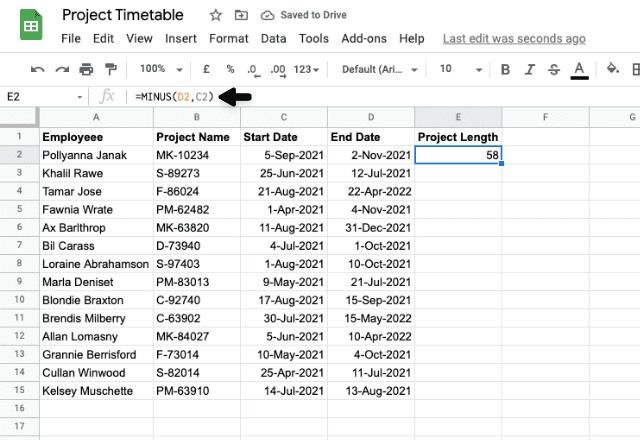#### Option 2: The DAYS formula

The DAYS function is a slightly smarter function you can use to subtract dates in Google Sheets. Not only does it return the total number of days between dates, but also factors in leap years. Here is the DAYS function formula:

=DAYS([end_date],[start_date])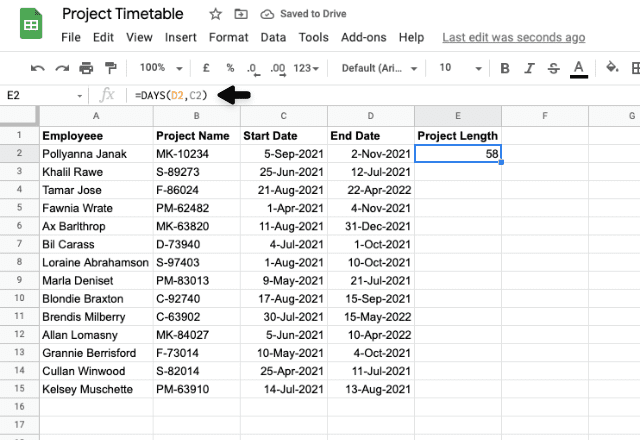Work in a finance role and need to calculate financial interest and fixed-income securities based on a 360-day year? Read our article on How to use the specialist function DAYS360.

The above options were the more basic ways you could calculate between days. The following options are more advanced, however, they leave you with more flexibility in your calculations.

In our example, the projects start and end on specific dates. But, team members will only work on the project on their work days, i.e. weekdays. Let’s take a look at these more advanced options to help us account for these variables.

#### Option 3: Use the NETWORKDAYS function

NETWORKDAYS is a very handy formula to subtract dates within Google Sheets whilst only counting weekdays. This formula stands for Net Working Days, thus excluding the weekends from its calculations.

Moreover, you can customize NETWORKDAYS to calculate non-standard working weeks (i.e. not Monday through Friday) or even to exclude public holidays. If you would like to learn more about this, check out our Guide to using the NETWORKDAYS function.

For this example, we will use the most simple syntax for NETWORKDAYS:

=NETWORKDAYS([start_date],[end_date])

For more customizable formulas, take a look at the NETWORKDAYS syntax.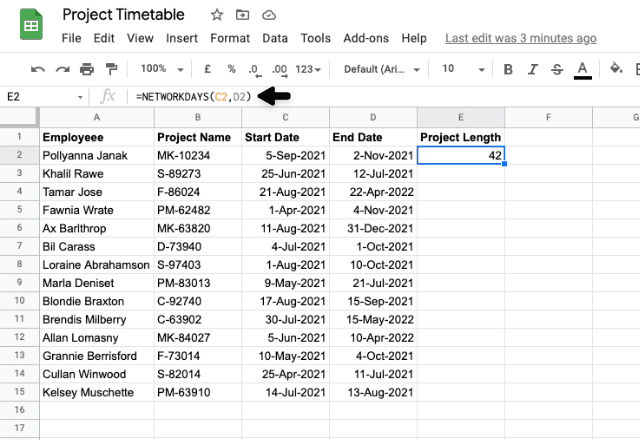NETWORKDAYS can successfully calculate the total number of working days. But what about if we want to calculate a date difference in months or years? The next option is the perfect solution.

#### Option 4: Use the DATEDIF function

DATEDIF stands for Date Difference. It allows you to calculate the difference in dates in either days, months or years.

Here is the basic DATEDIF syntax:

=DATEDIF([start_date],[end_date], unit)

The unit is the time measurement you want to use to calculate your date difference. Here is a comprehensive list of each unit and what it does: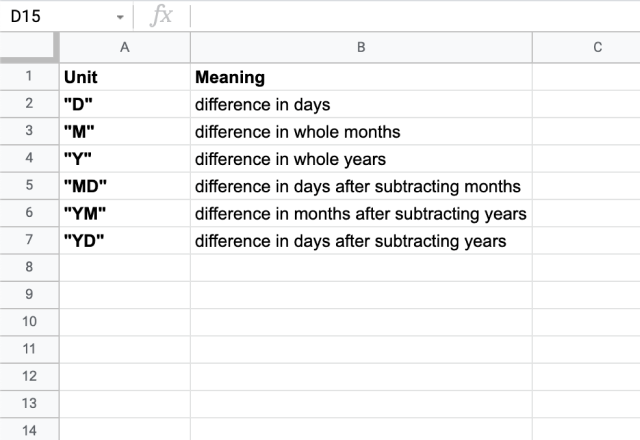Here are the examples using the three more basic DATEDIF formulas: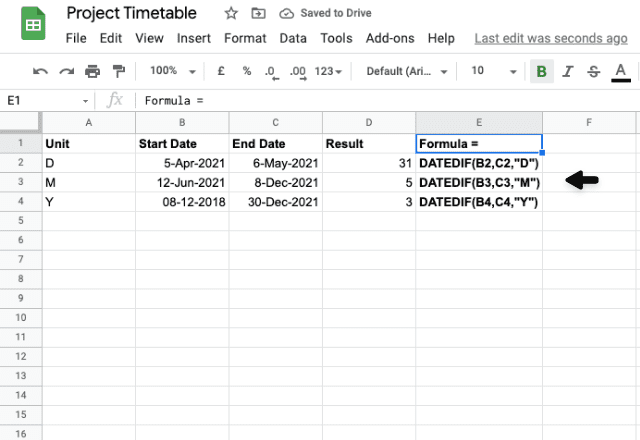As you can see, we have calculated the difference in dates using days, months and years as the measurement. The days formula essentially works the same way as the MINUS formula. Nevertheless, DATEDIF is the only formula that can achieve these calculations using months and years as the measurement units.

Now let’s look at the other DATEDIF formulas that you can use in Google Sheets.

##### How to use MD, YM and YD units in DATEDIF

Observing the unit list above, MD, YM and YD find a difference between dates by calculating one unit and excluding the other. Let’s look at some examples and see how each formula works.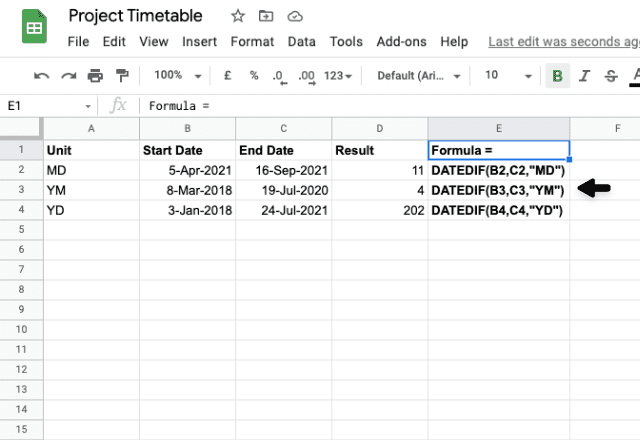As you can see, using the “MD” unit, the formula excludes the number of months in the calculation. Instead, it focuses on the difference between the two days (5th and 16th). Subtracting these from one another comes to 11.

For the “YM” unit, the formula excludes the difference in years between the two dates. It calculates the difference between March and July, which is 4.

Finally, calculating the date difference with “YD”, the calculation excludes the difference in years. It calculates the difference between 3 January and 24 July instead, which gives us 202.

If you would like a more in-depth breakdown of this formula, take a look at our page on DATEDIF syntax.

### And there you have it!

As you can see, there are numerous ways to calculate the difference between two dates in Google Sheets. Each formula offers a unique feature to help fulfill your objectives.